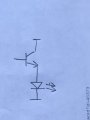# TL 431a voltage regulator circuit

Joined Sep 28, 2021
266
Hi all, below is a TL 431a voltage regulator circuit. Just out of curiosity, what can I do to replace TL 431a with its internal discrete components circuit so that I can better understand how a voltage regulator worksI have posted my LT Spice file below so that you can unzip and simulate it#### Attachments

• 3.5 KB Views: 1

#### MrSalts

Joined Apr 2, 2020
2,767
Look up,the datasheet of TL431a - a conceptual schematic of the internals are commonly shown in a datasheet.

#### crutschow

Joined Mar 14, 2008
33,346
I can better understand how a voltage regulator works
The basics are fairly simple.
When the TL431 REF input goes slightly above its 2.5V internal reference voltage it conducts more current from its cathode to anode.
If the REF input goes slightly below 2.5V, it conducts less current from cathode to anode.

Thus, in your posted circuit, the TL431 adjusts Q1's base current to keep the output at a voltage that provides 2.5V at the R2-R3 voltage divider output.

For R2 = 1kΩ and R3 = 3.6kΩ, the output voltage will be 3.19V to give 2.5V at the junction of R2 and R3.

If you wanted 5V out, for example, then you would increase R2 to 3.6kΩ (of course V1 would need to be some above 5V for that to work).

Note that the maximum cathode-anode current of the TL431 is 150mA, so you likely want to increase the value of R1 so that current is no more that 100mA.

•#### MrSalts

Joined Apr 2, 2020
2,767
If your simulator shows an output not equal to 2.5v, adjust the values of the 2.4K or 7.2k resistors. The ratio determines the output voltage. Drastic changes may not work and you can play with the exact values to dial in an "accurate" 2.500v but that value will shift some mV if you change the current limiting resistor that supplies power to your simulated LT431.

•#### Ian0

Joined Aug 7, 2020
8,942
If you are trying to understand how the circuit works, think of the TL431 as being like a transistor with a red LED in its emitter.The transistor starts to turn on when the adj (base) voltage exceeds Vbe of the transistor PLUS the LED voltage (about 2.5V in total).
So, as the output voltage increases, the voltage on adj increases. Then it reaches the threshold, the TL431 diverts current away from the 2N2222, reducing the voltage on its base, and consequently reducing the voltage on its emitter.
By the way, R1 is FAR too small. There would be too much current in the TL431. Try with 1k.

You can make the transistor-LED circuit - it will actually work, but won't be terribly accurate. You can also see how much current it is diverting from the 2N2222 by how bright the LED glows.

••